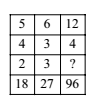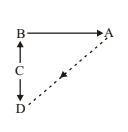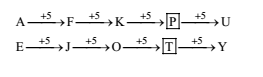# RRB ALP 2018 Practice Test Papers | Reasoning Questions (Day-26)

Dear Aspirants, Here we have given the Important RRB ALP & Technicians Exam 2018 Practice Test Papers. Candidates those who are preparing for RRB ALP 2018 can practice these Reasoning Questions to get more confidence to Crack RRB 2018 Examination.

[WpProQuiz 1913]

Click “Start Quiz” to attend these Questions and view Solutions

1. Find the missing number from the given responses:(a) 5

(b) 8

(c) 7

(d) 6

1. In the following question, select odd letters from the given alternatives.

(a) FUGT

(b) KPLO

(c) DWEV

(d) CWDX

1. DIRECTION: Select the related word from given alternatives.

hive: bee:: eyrie:?

(a) Pigeon

(b) Sparrow

(c) Parrot

(d) Eagle

1. Some equations are solved on the basis of a certain system. Find the correct answer for the unsolved equation on that basis.

5 * 6 = 35, 8 * 4 = 28, 6 * 8 =?

(a) 46

(b) 34

(c) 23

(d) 38

1. A is in the east of B which is in the North of C. If D is in the South of C., then in which direction of A, is D.

(a) North – West

(b) South

(c) East – East

(d) South–West

1. Identify the hidden name of the geometrical figure in the given word from among the answer words whose letters are given in jumbled form. SPIRITUAL

(a) RISPAL

(b) RITUAL

(c) SPIRIT

(d) RIPITU

1. Which will appear third in the dictionary?

(a) Monarchy

(b) Monastic

(c) Monetary

(d) Moneyed

1. AE, FJ, KO,? UY

(a) QN

(b) TQ

(c) NP

(d) PT

1. Water: Ocean:: Sand:?

(a) Island

(b) Waves

(c) River

(d) Desert

1. Find the wrong number in the series:

28, 33, 31, 36, 34, 29

(a) 33

(b) 36

(c) 34

(d) 29

5 + 4 = 9 and 9 × 2 = 18 6 + 3 = 9 and 9 × 3 = 27 12 + 4 = 16 and? = 96/16= 6

Except CWDX, All others have vowel.

A hive is a shelter for bees. Whereas, Aeyrie is a large nest of an eagle.

1. Answer: (a)RISPAL ® SPIRAL

The correct order is: Monarchy, Monastic, Monetary, Moneyed.

1. Answer: (d)Ocean is the mass of water

Similarly, Desert is the mass of sand.

28 + 3 = 31 &

36 + 3 = 39

33 + 3 = 36

So, wrong no. is 29.

RRB ALP 2018 Practice Test Papers | Reasoning Questions (Day-1)

RRB ALP 2018 Practice Test Papers | Reasoning Questions (Day-2)

RRB ALP 2018 Practice Test Papers | Reasoning Questions (Day-3)

RRB ALP 2018 Practice Test Papers | Reasoning Questions (Day-4)

RRB ALP 2018 Practice Test Papers | Reasoning Questions (Day-5)

RRB ALP 2018 Practice Test Papers | Reasoning Questions (Day-6)

RRB ALP 2018 Practice Test Papers | Reasoning Questions (Day-7)

RRB ALP 2018 Practice Test Papers | Reasoning Questions (Day-8)

RRB ALP 2018 Practice Test Papers | Reasoning Questions (Day-9)

RRB ALP 2018 Practice Test Papers | Reasoning Questions (Day-10)

RRB ALP 2018 Practice Test Papers | Reasoning Questions (Day-11)

RRB ALP 2018 Practice Test Papers | Reasoning Questions (Day-12)

RRB ALP 2018 Practice Test Papers | Reasoning Questions (Day-13)

RRB ALP 2018 Practice Test Papers | Reasoning Questions (Day-14)

RRB ALP 2018 Practice Test Papers | Reasoning Questions (Day-15)

RRB ALP 2018 Practice Test Papers | Reasoning Questions (Day-16)

RRB ALP 2018 Practice Test Papers | Reasoning Questions (Day-17)

RRB ALP 2018 Practice Test Papers | Reasoning Questions (Day-18)

RRB ALP 2018 Practice Test Papers | Reasoning Questions (Day-19)

RRB ALP 2018 Practice Test Papers | Reasoning Questions (Day-20)

RRB ALP 2018 Practice Test Papers | Reasoning Questions (Day-21)

RRB ALP 2018 Practice Test Papers | Reasoning Questions (Day-22)

RRB ALP 2018 Practice Test Papers | Reasoning Questions (Day-23)

RRB ALP 2018 Practice Test Papers | Reasoning Questions (Day-24)

RRB ALP 2018 Practice Test Papers | Reasoning Questions (Day-25)